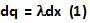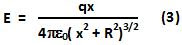## Monday, January 25, 2010

### Irodov Problem 3.11Consider an infinitesimally small section of the wire of length dx. The charge in this infinitesimally small section of wire will be,The force acting on this infinitesimally small section of the wire due to the ring will be,The electric field E due to the ring at a distance x along the axis has already been derived in the solution to problem 3.9 in Eqn 4 as,The total force acting on the wire is thus given by,